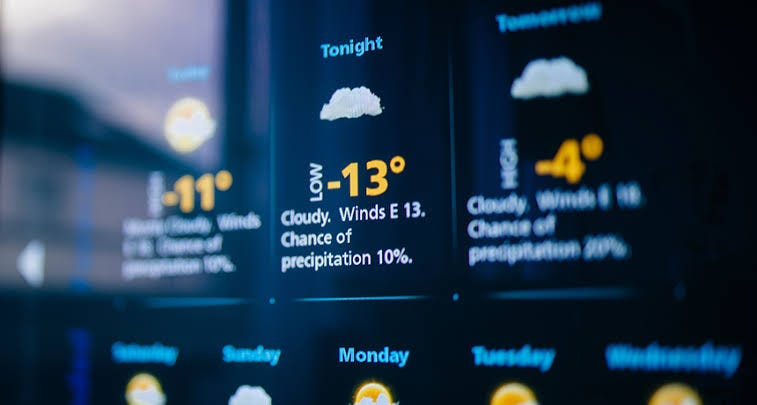# Weather forecasting with Recurrent Neural Networks

Source: Deep Learning on MediumWith advances in Data Science and Technology, we are able to get precise forecasts of the weather in almost every location around the world. Data collected from weather stations and satellites are used to analyze and predict the meteorological disasters caused by extreme weather. Machine Learning can be used to forecast weather with historical weather data.

In this article, we will develop a deep learning model with Recurrent Neural Networks to provide 4 days forecast of the temperature of a location by considering 90 days of historical temperature data.

# What is Recurrent neural network(RNN)?

RNN is a deep learning model that is used for Time-series prediction etc. RNN is used when we want to predict a future outcome based on the previous inputs. For example, we can use RNN to predict the next word in a sentence by providing previous words.

# RNN for Weather forecasting.

We will develop an RNN model that can provide 4 days forecast of temperature based on 30 days of historical temperature data.

Let’s load the dataset and see the first few rows:

`import numpy as npimport matplotlib.pyplot as pltimport pandas as pd#import dataset from data.csv filedataset = pd.read_csv('data.csv')dataset = dataset.dropna(subset=["Temperature"])dataset=dataset.reset_index(drop=True)training_set = dataset.iloc[:,4:5].values`